# How to calculate days between dates in Excel

This tutorial will teach you a few quick and easy ways to find out how many days are between two dates in Excel.

Are you wondering how many days between two dates? Maybe, you need to know the number of days between today and some date in the past or future? Or, you just want to count working days between two dates? Whatever your problem is, one of the below examples will certainly provide a solution.

## Days between dates calculator

If you are looking for a quick answer, just supply the two dates in the corresponding cells, and our online calculator will show you how many days there are from date to date:

Curious to know the formula that has calculated your dates? It's as simple as `=B3-B2` :)

Below you will find the detailed explanation on how this formula works and learn a few other methods to calculate days between dates in Excel.

## How many days between dates calculation

The easiest way to calculate days between dates in Excel is by subtracting one date from another:

For example, to find out how many days are between dates in cells A2 and B2, you use this formula:

`=B2 - A2`

Where A2 is an earlier date, and B2 is a later date.

The result is an integer that represents no. of days between two dates: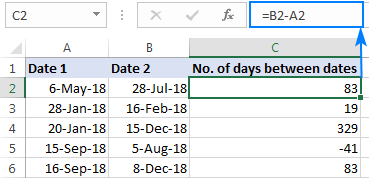### How this formula works

As you probably know, Microsoft Excel stores dates as serial numbers starting on 1-Jan-1900, which is represented by the number 1. In this system, 2-Jan-1900 is stored as the number 2, 3-Jan-1900 as 3, and so on. So, when subtracting one date from another, you actually subtract the integers representing those dates.

In our example, the formula in C3, subtracts 43226 (the numeric value of 6-May-18) from 43309 (the numeric value of 28-Jul-18) and returns a result of 83 days.

The beauty of this method is that it works perfectly in all cases, no matter which date is older and which is newer. If you are subtracting a later date from an earlier date, like in row 5 in the screenshot above, the formula returns a difference as a negative number.

## Calculate number of days between dates in Excel with DATEDIF

Another way to count days between dates in Excel is by using the DATEDIF function, which is specially designed to work out the date difference in various units, including days, months and years.

To get the number of days between 2 dates, you supply the start date in the first argument, end date in the second argument, and "d" unit in the last argument:

DATEDIF(start_date, end_date, "d")

In our example, the formula goes as follows:

`=DATEDIF(A2, B2, "d")`

Unlike the subtraction operation, a DATEDIF formula can only subtract an older date from a newer date, but not the other way round. If the start date is later than the end date, the formula throws a #NUM! error, like in row 5 in the screenshot below: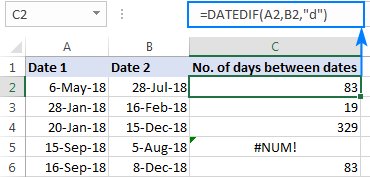Note. DATEDIF is an undocumented function, meaning it is not present in the list of functions in Excel. To build a DATEDIF formula in your worksheet, you will have to type all the arguments manually.

## Count days between dates with Excel DAYS function

The users of Excel 2013 and Excel 2016 have one more amazingly simple way to calculate days between two dates - the DAYS function.

Please pay attention that compared to DATEDIF, a DAYS formula requires the arguments in the reverse order:

DAYS(end_date, start_date)

So, our formula takes the following shape:

`=DAYS(B2, A2)`

Like subtraction, it returns the difference as a positive or negative number, depending on whether the end date is greater or smaller than the start date: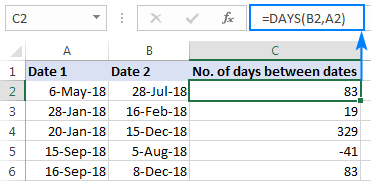## How to calculate number of days between today and another date

In fact, calculating the number of days from or before a certain date is a particular case of "how many days between dates" math. For this, you can use any of the formulas discussed above and supply the TODAY function instead of one of the dates.

To calculate the number of days since date, i.e. between a past date and today:

TODAY() - past_date

To count the number of days until date, i.e. between a future date and today:

Future_date - TODAY()

As an example, let's calculate the difference between today and an earlier date in A4:

`=TODAY() - A4`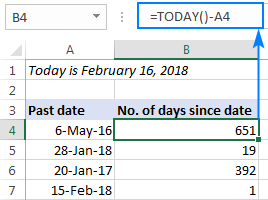And now, let's find out how many days are between today and a later date: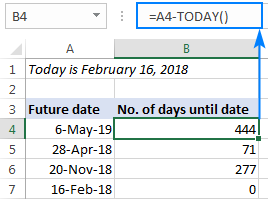## How to calculate working days between two dates in Excel

In situations when you need to get the number of days between two dates without weekends, use the NETWORKDAYS function:

NETWORKDAYS(start_date, end_date, [holidays])

The first two arguments should already look familiar to you, and the third (optional) argument allows excluding a custom list of holidays from the day count.

To find out how many working days are between two dates in columns A and B, use this formula:

`=NETWORKDAYS(A2, B2)`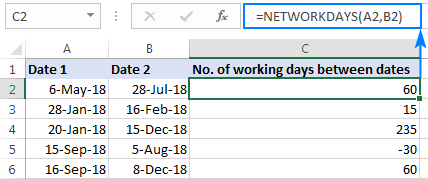Optionally, you can enter your holiday list in some cells and tell the formula to leave out those days:

`=NETWORKDAYS(A2, B2, \$A\$9:\$A\$10)`

As the result, only business days between two dates are counted: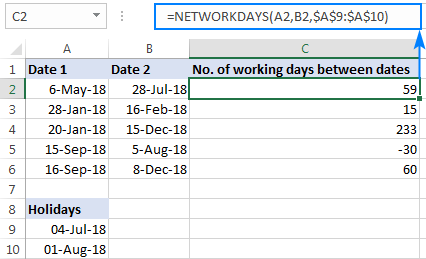Tip. In case you need to handle custom weekends (e.g. weekends are Sunday and Monday or Sunday only), use the NETWORKDAYS.INTL function, which allows you to specify what days of the week should be considered weekends.

## Find number of days between two dates with Date & Time Wizard

As you see, Microsoft Excel provides a handful of different ways to count days between dates. If you are not sure which formula to use, let our Date & Time Wizard do the how-many-days-between-two-dates calculation for you. Here's how:

1. Select the cell in which you want to insert the formula.
2. On the Ablebits Tools tab, in the Date & Time group, click Date & Time Wizard: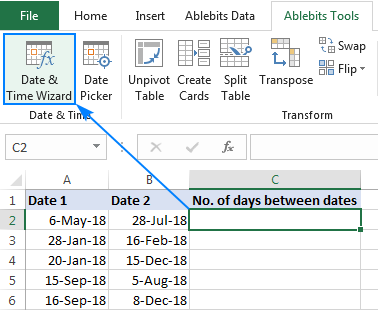3. In the Date & Time Wizard dialog window, switch to the Difference tab and do the following:
• In the Date 1 box, enter the first date (start date) or a reference to the cell containing it.
• In the Date 2 box, enter the second date (end date).
• In the Difference in box, select D.

The wizard immediately shows a formula preview in the cell and the result in the Difference in box.

4. Click the Insert formula button and have the formula inserted in the selected cell. Done!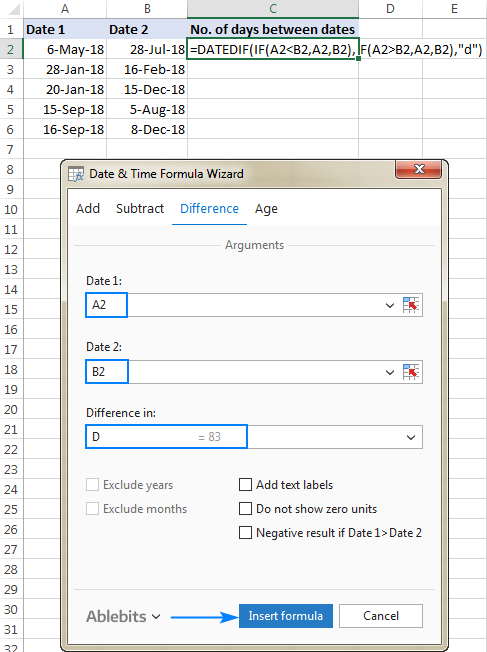A double-click on the fill handle, and the formula gets copied across the column:To display the date difference in a slightly different way, you are free to choose any of the additional options:

• Show text labels - the word "days" will appear along with the number, like shown in the screenshot below.
• Do not show zero units­ - if the date difference is 0 days, an empty string (blank cell) will be returned.
• Negative result if Date 1 > Date 2 - the formula will return a negative number is the start date is later than the end date.

The screenshot below shows a couple of additional options in action: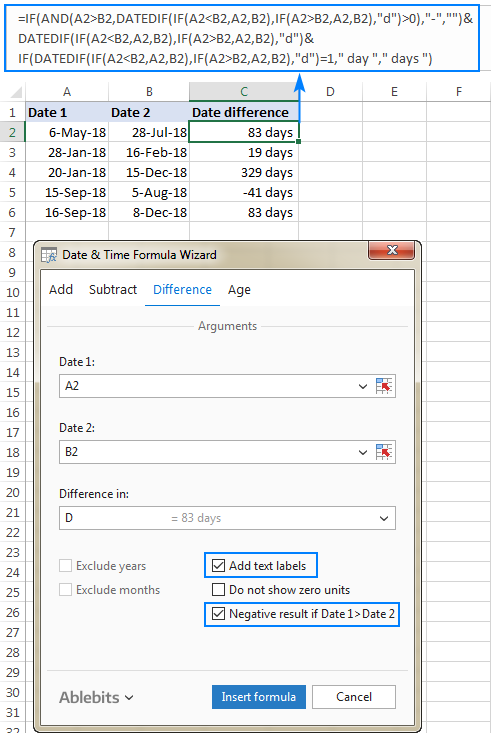This is how you calculate the number of days between dates in Excel. If you'd like to test our Date & Time Formula Wizard in your worksheets, you are welcome to download 14-day trial version of Ultimate Suite, which includes this as well as 70+ other time-saving tools for Excel.

How Many Days Between Dates - examples (.xlsx file)

## You may also be interested in

1. how to calculate days between two dates i.e. suppose 16/7/2023 is the end date of fiscal year and 14/04/2023 is the start date. Further, if we cross the end date, the number of days will be of color red? how will we do that

2. HI and Thank you for sharing this

It is work fine but i faced an issue

when I calculate the days between 23/07/2023 and 31/07/2023 will return 6 but the correct result is 7 days,

note: i faced in different dates as well

Thanks

• The correct result is 8 days, if you don't use the time. This is July 23,24,25,26,27,28,29,30.

• No the correct result is 7 if you count it from the calendar (normal count). with out weekend days

but if I used your formal the result will show me 6 days and that not correct.
but the same formal I used in different days [03 July 2023 t0 13 july 2023] without weekend days the count of days will be 9, and that correct

but why in the first one not correct, and i am using the same formal.

I hope you get my point.

Thanks

• Your previous question didn't say anything about weekends. Also, I can't know what formula you use and what days are counted as weekends. However, we have written many times here that a date without a time is implied at the beginning of the day. For example, your dates are 23.07.23 00:00:00 to 31.07.23 00:00:00.

3. HI, what is the formula to Return the last year of the given dates?
E.g.
19 May 2022 to 31 Dec 2022
19 Jan 2021 to 31 Dec 2021

• Hi! If I understand the question correctly, use the DATE function to create a new date
Try this formula:
=DATE(YEAR(A1),12,31)

4. Hello,
The question I have is related to dates in Excel since I am trying to find the difference between the two dates. Here are some examples: Concerning item A, everything is clear, as I used datedif, days, and the normal B1-A1 formula. Item B has an error because the days and months are different from Item A. Item B will be changed like item A, so I need to modify with formula 12/05/2026 to 05/12/2026 using a formula. Could you please assist in this issue?

Start End Diff.
A) 04/24/2026 05/26/2026 32
B) 12/05/2026 14/08/2026 -

Thanks

• Hi! Use substring functions to extract numbers from text. Use the DATE function to create the desired date.
Try this formula:

=IF(ISTEXT(A1),DATE(RIGHT(A1,4),LEFT(A1,SEARCH("/",A1)-1),MID(A1,SEARCH("/",A1)+1,SEARCH("/",A1,4)-SEARCH("/",A1)-1)),A1)

5. Need some help with this concern... thank you in advance

Leave is between Jan 01-2023 Until Jan 10-2023

there is a weekly report stating how many leaves done in a weekly basis

Week start Jan 02 - result should be 5 days not counting 2 days as literally it is an OFF
Week start Jan 09 - result should be 2 days

above item is just the rationale....

the real data is

Employee 1 - Leave between Dec 30 2022 until June 1 2023
Employee 2 - Leave between Jan 1, 2023 until Jan 10, 2023
Employee 3 - Leave between Jan 6, 2023 until Jan 11, 2023

Result should be
WS Jan 02 - 13 days
WS Jan 09 - 10 days

note cap days is 5 days only

Wish you could help me this one

• Hi!
To count the number of days excluding weekends, use this guide: Calculating weekdays in Excel - WORKDAY and NETWORKDAYS functions.

=NETWORKDAYS(MAX(A1,\$D\$1,0)*(A1<\$D\$1+6), MIN(B1,\$D\$1+6)*((MAX(A1,\$D\$1,0)*(A1<\$D\$1+6))>0))

A1, B1 - start and end date for Employee 1. D1 - week start date

6. Greetings,

I need help on excel formula to calculating penalty below.

There is penalty of 1% of the tax due for failure to file a return on time plus an additional 1%
for each thirty days the return is late. The minimum penalty for late payment is \$5.00

Thanks

• Hello!
To determine how many times a period of thirty days has been exceeded, use the MOD function -

=((B1-A1)-MOD(B1-A1,30))/30

7. In case start date and end date is same then how will we calculate the day

• Hi!
Add 1 to the day difference.

8. Hello! First of all, thank you. As an Excel newbie, I find all of your tutorials very helpful. If you would be so kind as to let me know if there is a formula for my problem. My boss wants the total absences for each month totaled. However, the way the workbook is set out is "start date" and "end date". My problem is some absences' end date crossed another month.

Type Start Date End DateTotal Days
Sick Leave Apr-12 Apr-13 2
Sick Leave Apr-30 May-02 3

• Hello!
Add another column D with the formula

=MIN(C1,EOMONTH(B1,0))

The EOMONTH function will determine the last day of the month.
Find the difference in days between columns D and A as described in the article above.

9. Hello,

I am wanting to work out the number of days between dates and clearly this is the right place.
I have followed the simple suggestion of minus one date cell from the other and this, for example, calculates 1 day between 27 and 28 March 2022.
However, with my job, I class this as 2 days. I start work on 27 March and leave on the 28 and charge 2 full days rate.
How do I ask Excel to see this as 2 days.
Another example would be 09 to 24 January 2023 where Excel is showing 15 days when I need it to calculate 16 days

• Hi!

10. How do I calculate the number of days between 2 dates if the dates are in this number format: 11/12/2021 and 04/01/2022? It seems Excel can only do it if the format is either like this: 2021/12/11 or 11-Dec-2021

11. Hi there,

I need help calculating the difference between two dates. I need to find out how many days a patient has been in our facility based on the entry /discharge dates within the month of November 2021 for example. Below are the entry/discharge dates and the number of days I need to obtain.Where discharge day is blank means the pacient is still in the facility so I need to use the month end date.
Any help/comment is much appreciated. Many thanks.

Beginning period End period
1/11/2021 30/11/2021

Entry date Discharge date No of days
1/10/2021 30
16/11/2021 21/12/2021 15
3/11/2021 26/11/2021 23
1/11/2021 30
28/09/2021 15/11/2021 14

• Hi!
To get the last day of the month uses the EOMONTH function.

=IF(B1="",EOMONTH(A1,0)-A1,B1-A1)

However, I do not really understand how between November 16 and December 21 you get 15 days.

12. Hi,

Now I have another Issue. That is, I need to find the days after the days crossed (for example: I entered one employee passport date of expiry 15/05/2020 and after the expiry date the days are not counting)

can you please clarify this also...

And your formula works, but I need to find also the days after expiry (i.e days with - )

• Hi!

=IF(A1 < TODAY(),TODAY()-A1,"")
or
=IF(A1 < TODAY(),TODAY()-A1,A1-TODAY())

Depending on what result you want to get.

• Hi

I have tried your formula but its not what I ask. So i have shared you my sample excel with you. If possible please clarify.

Actually I need all in one cell like If I enter past date, todays date or upcoming dates. I need to get result.

=IF(A1 < TODAY(),TODAY()-A1,A1-TODAY()) This formula it is not showing the past dates result in (Minus)

For Example

Passport Expiry - 25/08/2019
The result coming - 975 days (by using your formula)

But I need result - (-975 days)

if Passport Expiry - 25/08/2022
The result - 121 days (this is ok)

So I need the past dates result in minus (-)

Hope you understand my question. Please clarify

Thanks

• Hi,

Sorry the below result is also not correct.

if Passport Expiry - 25/08/2022
The result - 121 days (this is also not correct)

Thanks

• Hi!
Just make the result a negative number -

=IF(A1 < TODAY(),-(TODAY()-A1),A1-TODAY())

You can use a custom number format

#;"(-"#")";0

• Hi,

Thanks for your help. It working now but still I can see the cell with (-44678). But its ok... Thanks again

13. Hi,

I would like to calculate the remaining days with the current date (TODAYS DATE). So I use formula (=upcoming date - TODAY()). I get the result. But if I am coping same formula to the other cells which is "upcoming date cell is empty" the result showing "-44673" . But I need the cell to be empty or 0 instead of showing this "-44673". Is there any solution Please help me out.

Thanks

• Hi!
For conditional calculations, use the IF function:

=IF(A1>TODAY(),A1-TODAY(),"")

• Hi,

Thanks for your reply and Yes I got my answer... Its perfect. Thanks again for help me out...

• Hi,

Now I have another Issue. That is, I need to find the days after the days crossed (for example: I entered one employee passport date of expiry 15/05/2020 and after the expiry date the days are not counting)

can you please clarify this also...

And your formula works, but I need to find also the days after expiry (i.e days with - )

14. How many days Between 3/1/22 to 3/28/22

where, Holidays: 3/19/2, 3/26/22
Weekend: Friday Only
Absent Days: 3/3/22, 3/17/22

Please Give Solution With Details Formula

• Hi!
Please check out this article to learn how to count weekdays between 2 dates with custom weekends. Use NETWORKDAYS.INTL function.

15. Hi
I want to find the number of days from a date till today, the issue i am facing is the date format available is mmddyy but system date format is mmddyy hence i am getting value error, plz help

16. Hello Team,

Greeting,
Could you please assist me on how to find the number of days between dates that includes hours.

(A) Date of arrival: 01-March-2022 05:30
(B) Date of departure: 08-March-2022 21:45

what is the total number of days between A to B

• Hello Sir!
I'd like to ask you how to calculate total days, hour, and minutes between 2 cells that both used =int formula. Is there any formula that can be used to find the sum of those 2 cells? Thank you in advance!

• Hi!
I’m not sure I got you right since the description you provided is not entirely clear. Do you want to calculate the difference or sum of cells? What does the function INT have to do with it?
Have you tried using the formula right above your question?

• I'm sorry for the confusing question.
I will provide example then :)

I had 4 cells that contain dd/mm/yyyy hh:mm information and 2 cells that used INT function

A. Departure from X : 07/03/2022 18:00
B. Arrival at Y : 09/3/2022 19:30
C. Total number of days and hours using INT function like this
=INT(F13-E13)& "days, "&HOUR(F13-E13) & "hours, "&MINUTE(F13-E13) & " minutes"
Result : 2 days, 0 hours, 30 minutes

D. Departure from Y : 11/03/2022 07:10
E. Arrival at Z : 12/03/2022 14:30
F. Total number of days and hours using INT function
=INT(I13-H13)& "days, "&HOUR(I13-H13) & "hours, "&MINUTE(I13-H13) & " minutes"
Result : 1 days, 7 hours, 20 minutes

I'd like to know if there is formula I can use to calculate total number of days for trip from X to Z based on cell C and F. Usually I calculate it manually and type it to the Total Trip Duration cell.

Thank you :)

• Hi!
Based on cells C and A, you cannot calculate anything, since text is written there. Use cells A and E.

• I guess I have to calculate it manually. I think using cells A and E will result total days of the trip from the start to the end without subtracting the rest days (stops at Y). It will result 4 days 20 hours 30 minutes, instead of 3 days 7 hours 50 minutes.

Have a good day! :)

• Hi I ask you a question

In my excel sheet I have two dates 26/7/2023 and 27/7/2023 I want to display one date what a formula for it

17. Hello,

Is there a formula that can be used to calculate the total number of days for multiple date ranges in a table? I want to to avoid creating a new column (Total Days) and then adding the total number of days together to get the grand total.

Sample Data:

Start Date End Date Total Days
11/9/2018 12/5/2018 26
12/7/2018 12/25/2018 18
12/10/2018 12/30/2018 20

Grand Total days = 64

• Hello!
You can sum in one formula several values calculated using the DAYS or DATEDIF functions

=DAYS(B2, A2) + DAYS(B3, A3) + DAYS(B4, A4)

18. I'm trying to workout number of days within a period and are within a period.
for example Start Date 01/01/2022, End Date 31/06/2022, however i only want to know the days within 02/2022 within the intial period.

Anyhelp from anyone

• Hi!
Sorry, it's not quite clear what you are trying to achieve. Do you want to get a list of days? Or do you need to find the sum for these days of February? Or count the number of days?

• I really wanna know how to solve this with excel formula as well, thanks

• Hi!
Can you explain exactly and clearly what you want to know?

19. Hi Sir,

I need to find the time period 00:00 to 08:00 hrs from two dates with time, for example 12/01/22 22:00 to 14/01/22 18:00. I would like to know how many times the period 00:00 to 08:00 occurred in this date range and specifically on which dates.

20. HOW TO DAYS CALCULATION DATE 25.06.21 TO 08.09.21

• Hi!
The answer is in the article above.

21. I am trying to calculate the number of days between 2 dates, ensure I am including leap year thinking plus a cap at 3 years
start date end date
06/26/1989 07/19/2021 = 11711 days =DAYS(B4-A4)

end date
07/19/2021 - (minus) = new date
how can i cap this from going back further than 3 years? 07/19/2018
does this formula also include the extra day when a leap year is included?

• Hi!
Pay attention to the following paragraph of the article above - Count days between dates with Excel DAYS function

22. Need a formula to show the percentage reminding for a task if the start date in column A = 7/3/2021 and the due date in column B is 12/23/2021. Need to place % it in column D.

Thanks

23. I Have A question suppose in a cell I want subtraction of two dates and result comes Zero Day or 1 Day or 2 Days or Minus 1 Day or Minus 2 Days. How do the cells show Day or Days including the Numbers as mentioned?

24. Formulla

no. Of days between two dates when end date becomes first date.

25. days between 1-4-2020 to 31-3-2021 is 365. when we use datedif , it comes 364

• Hello!
The calculation is correct, since the date 31-3-2021 means 31-3-2021 00:00:00. This day is not included in your date range.

• Is there a way to include the start date and end date in the calculation?

• Hi!